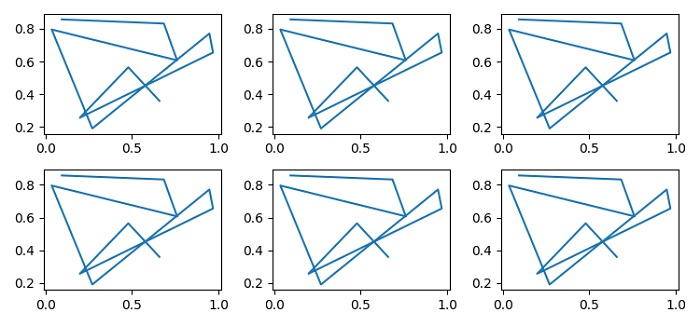# What does axes.flat in Matplotlib do?

Axes.flat means a 1D iterator over the array. Let's take an example to see how to use axes.flat.

## Steps

• Set the figure size and adjust the padding between and around the subplots.

• Create a figure and a set of subplots using subplots() method.

• Create x and y data points using numpy.

• Use axes.flat and iterate all the axes (step 2).

• Plot x and y data points using plot() method.

• To display the figure, use show() method.

## Example

import numpy as np
from matplotlib import pyplot as plt

plt.rcParams["figure.figsize"] = [7.50, 3.50]
plt.rcParams["figure.autolayout"] = True
fig, axes = plt.subplots(nrows=2, ncols=3)
x = np.random.rand(10)
y = np.random.rand(10)
for _, ax in enumerate(axes.flat):
ax.plot(x, y)
plt.show()

## Output